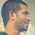# System Analysis and Design Set 18

## Questions 171 to 180

 171 The main issue in documentation during the __________ phase is to determine what the system must do. (a)  Analysis                                                          (b)  Design (c)  Implementation                                              (d)  Coding                    (e)  Maintenance. 172 the symbol represents (a)  Aggregation                                                   (b)  Generalization        (c)  Dependency                                                   (d)  Composition           (e)  Association. 173 _____ design is a data-flow-based methodology. (a)  Physical                   (b)  Logical                    (c)  Structure                 (d)  Object Oriented     (e)  Record based. 174 Which of the following UML diagram provides static structure of a system? (a)  Class diagram                                                 (b)  Sequence diagram (c)  State diagram                                                  (d)  Collaboration diagram (e)  Activity diagram. 175 System Testing implies_______________ (a)     Testing changes made in an existing or a new program (b)    Making sure that the new programs do in fact process certain transactions according to specifications (c)     Running the system with live data by the actual user (d)     Executing a program to check logic changes made in it and with the intention of finding errors   making the program fail (e)     Both (a) and (b) above. 176 _________refers to the number of connections between a “calling” and “called” module and the complexity of these connections. (a)  Module coupling                                           (b)  Transaction (c)  Evaluation                                                       (d)  Cohesion                (e)  Collaboration. 177 Which of the following highlights the feasibility report of an existing system? (a)  Dataflow and processing                              (b)  Information needs (c)  Problem areas                                                 (d)  Data storage           (e)  All the above. 178 Which diagram depicts a pattern of messages coming into and going from an object? (a)  Collaboration diagram                                   (b)  Sequence diagram (c)  Class diagram                                                 (d)  Object diagram                    (e)  Activity diagram. 179 The process of converting a new or revised system design into an operational one is known as _______________________. (a)  Testing                                                            (b)  Implementation (c)  Quality Assurance                                            (d)  System Analysis                 (e)  System Design. 180 The rules and semantics of the UML can be expressed in a form known as (a)  Object modeling language                            (b)  Object constraint language (c)  Object specification language                     (d)  Object control language (e)  Object driven language.

<< PrevNext >>

1.2.